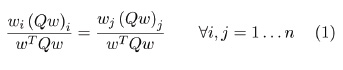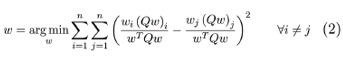# Risk-Parity Strategies For Equity Portfolio Management

April 05, 2014Risk-based strategies have gained popularity amid market uncertainty, and many are now being touted as "smart beta," providing a systematic way to outperform traditional capitalization-weighted benchmarks. Here we examine the notion of risk parity, taking what has almost exclusively been discussed in an asset allocation context and applying its concepts to equity-only portfolio construction.

Risk parity seeks to equalize sources of risk such that the relative marginal contribution to risk (RMCTR) from each source is equal. Historically, research has treated asset classes as the sources of risk, because these are typically quite distinct and relatively uncorrelated (for example, interest rate versus equity market risk). Given an n-vector of loadings on sources of risk W and their covariance matrix Q, a risk parity portfolio satisfies:In a traditional asset allocation setting where n represents the number of asset classes, n is "small" (<10), and this problem is easy to solve, even using odd formulations and mediocre solvers. In equity portfolios, n is usually larger, and the sources of risk may be individual stocks, groupings of stocks (sectors, countries, regions or combinations thereof) or quantitative risk factors. By modeling risk parity as a set of optimization constraints, we are able to find risk-parity portfolios efficiently for large n. In addition, we will demonstrate how certain formulations of risk parity can in fact be used as an overlay on top of an existing investment process. The following discussion is divided into three parts. We begin with the simplest case, where the elements of w represent individual stocks, so each stock constitutes a source of risk (dubbed "asset risk parity" henceforth). Next, the analysis will expand to include risk parity between groupings of stocks (such as country/sector). Finally, we introduce the idea of risk parity between risk factors.

Rather than presenting a series of backtests with the goal of promoting risk-parity strategies and validating their possible superior performance, we discuss methods for constructing risk-parity portfolios and analyze how each variant of risk parity affects the resulting portfolio composition.

Constructing Asset Risk-Parity Portfolios

Despite its straightforward definition and prevalence in research, there is little discussion—let alone consensus—on exactly how risk-parity portfolios are built. Methods discussed include model simplification (e.g., ignoring correlations, per Anderson et al. (2012)), ad-hoc/trial-and-error approaches using rudimentary statistical software (Kazemi, 2012) and iterative numerical algorithms (Chaves et al. 2012). Finding a portfolio that satisfies Equation 1 is sometimes tackled by using a nonlinear optimizer to minimize a loss function measuring the portfolio's deviation from risk parity, for example, the sum of squared differences between stocks' RMCTR, as in Maillard et al. (2010):In the equity world where n is typically large, contrary to this conventional method, it is preferable to model risk parity as optimization constraints. In the case of a long-only portfolio where w represents individual stock weights, Equation 1 can be expressed as a set of convex constraints on the RMCTR of each stock:Here Λi is a diagonal matrix with Λii =1 and zero elsewhere, and n represents the total number of stocks. Equation 3 can be formulated and solved as a convex optimization problem. Additionally, when minimizing risk-parity violations using the objective function, per Equation 2, depending on the quality of the search algorithm, one may end up with portfolios that are in fact not risk parity but have simply exhausted that particular optimizer's ability to find a better solution. Finally, minimizing departure from risk parity is dangerous because depending on the loss function, it may be difficult to differentiate between large violations involving a few stocks and small violations across the board.